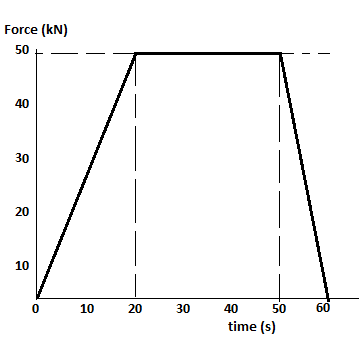# Passengers on a commercial jet experience changes in horizontal force as the jet accelerates and...

## Question:

Passengers on a commercial jet experience changes in horizontal force as the jet accelerates and decelerates in mid flight without changing altitude. The graph below shows the horizontal force versus time exerted on the 90000 kg jet as it accelerates from an initial velocity of 187.8 m/s to its final cruising velocity. According to the graph, what is the final cruising velocity of the jet? Show your work.## Impulse:

The sudden application of a force on a body which acts over a small time interval (dt) is termed as an impulse of the force on the body.

{eq}I = \int F. dt {/eq}

The impulse is also calculated using the change of momentum of the body within that small time interval.

## Answer and Explanation:

Become a Study.com member to unlock this answer! Create your account

In the graph, we see the variation of the horizontal force of the jet with time.

The area under the force-time graph gives us the change in momentum...

See full answer below.

#### Learn more about this topic:The Law of Conservation of Momentum

from

Chapter 13 / Lesson 2
58K

After completing this lesson, you will be able to explain what conservation of momentum is and use the equation for conservation of momentum to solve problems. A short quiz will follow.